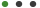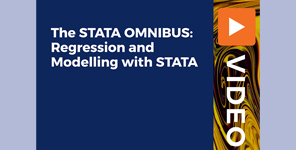### The STATA OMNIBUS: Regression and Modelling with STATAPackt Publishing

# The STATA OMNIBUS: Regression and Modelling with STATA

Get acc Titlewise MetadataClipwise MetadataSheet3 Exploreess to a fully updated course that simplifies the concept of linear and non-linear regression, regression modeling, and StataUpdated on Sep, 2023Language - EnglishPackt PublishingBusiness,Business Analytics & Intelligence,Regression Analysis30-days Money-Back Guarantee

Training 5 or more people ?

## Course Description

Learning and applying new statistical techniques can be a daunting experience, especially when working with complex datasets that do not allow for easy click-and-go analysis but require a deeper level of understanding of analytical methodologies.

This course will introduce you to data analytics using Stata and prepare you to handle real-life complex datasets with ease. Starting with an overview of the learning objectives, it takes you straight into the concepts of linear regression, non-linear regression, and regression modeling. Once you’ve got a comprehensive introduction to Stata and its various uses in modern data analysis, you’ll discover how you can use Stata extensively for manipulating, exploring, visualizing, and modeling complex types of data. As you advance, you’ll also learn how to use the Analysis of Variance (ANOVA) test to find out significant differences between different groups. You’ll then explore the functionality and application of ordinary least squares (OLS) and study how logit and probit models work. Finally, you’ll cover some simulation techniques and features of the count data model and get to grips with survival and panel data analysis.

By the end of this course, you’ll have developed the skills and ability to engage with Stata for handling complex data analytics.

All the code files and resources for this course are available at https://github.com/PacktPublishing/The-STATA-OMNIBUS-Regression-and-Modelling-with-STATA

### Goals

What will you learn in this course:

• Distinguish between linear and non-linear regression analysis
• Model non-linear relationships in linear regression
• Perform regression modeling and data visualization in Stata
• Interpret and analyze regression output from ordinary least squares (OLS)
• Understand the basic statistical intuition behind non-linear regression
• Work with dummy variables in logit and probit regression

### Prerequisites

What are the prerequisites for this course?

• There are no requirements except curiosity## Curriculum

Check out the detailed breakdown of what’s inside the course

Introduction
1 Lectures
•Introduction 02:58 02:58
Linear Regression
26 LecturesNon-Linear Regression
22 LecturesRegression Modelling
14 LecturesIntroduction to Stata – Getting Started
6 LecturesIntroduction to Stata – Exploring Data
7 LecturesIntroduction to Stata – Manipulating Data
13 LecturesIntroduction to Stata – Visualizing Data
13 LecturesIntroduction to Stata: Testing Means, Correlations, and Analysis of Variance (ANOVA)
4 LecturesIntroduction to Stata: Linear Regression
6 LecturesIntroduction to Stata: Categorical Choice Model
3 LecturesIntroduction to Stata: Random Numbers and Simulation
4 LecturesIntroduction to Stata: Count Data Models
5 LecturesIntroduction to Stata: Survival Analysis
7 LecturesIntroduction to Stata: Panel Data
6 Lectures## Instructor DetailsPackt Publishing

Founded in 2004 in Birmingham, UK, Packt's mission is to help the world put software to work in new ways, through the delivery of effective learning and information services to IT professionals.

Working towards that vision, we have published over 6,500 books and videos so far, providing IT professionals with the actionable knowledge they need to get the job done - whether that's specific learning on an emerging technology or optimizing key skills in more established tools.

As part of our mission, we have also awarded over \$1,000,000 through our Open Source Project Royalty scheme, helping numerous projects become household names along the way.

## Course Certificate

User your certification to make a career change or to advance in your current career. Salaries are among the highest in the world.